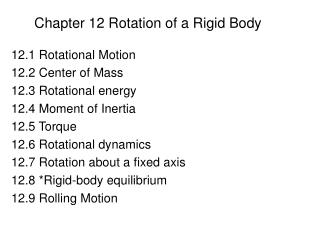DownloadDownload PresentationChapter 12 Rotation of a Rigid Body

# Chapter 12 Rotation of a Rigid Body

Download Presentation## Chapter 12 Rotation of a Rigid Body

- - - - - - - - - - - - - - - - - - - - - - - - - - - E N D - - - - - - - - - - - - - - - - - - - - - - - - - - -
##### Presentation Transcript

1. Chapter 12 Rotation of a Rigid Body 12.1 Rotational Motion 12.2 Center of Mass 12.3 Rotational energy 12.4 Moment of Inertia 12.5 Torque 12.6 Rotational dynamics 12.7 Rotation about a fixed axis 12.8 *Rigid-body equilibrium 12.9 Rolling Motion

2. Stop to think 12.1 page 342Stop to think 12.2 page 350Stop to think 12.3 page 353Stop to think 12.4 page 357Stop to think 12.5 page 364 • Example 12.2 page 343 • Example 12.5 page 348 • Example 12.7 page 349 • Example 12.14 page 359 • Example 12.16 page 361 • The great Downhill Race Page 395

3. Angular velocity and angular acceleration • Angular velocity • The sign convention is that ω is positive for counterclockwise (ccw) rotation, negative for clockwise (cw) rotation. • Angular acceleration Here α is angular acceleration

4. Angular velocity and acceleration • Question: • What is the angular speed of ladybug1 • What is the ratio of linear speed of ladybug2 to ladybug1 • What is the ratio of the centripetal • acceleration of ladybug2 to ladybug1

5. The sign of angular velocity and acceleration

6. The center of mass

7. A 500 g ball and a 2.0 kg ball are connected by a massless 50 cm-long rod. Where is the center of mass

8. Torque • The ability of a force to cause a rotation depends on three factors • (1) the magnitude F of the force • (2) the distance r from the point of application to the pivot • (3) The angle at which the force is applied

9. Quick quiz: The rods all have the same length and are pivoted at the dot. Rank in order, From largest to smallest, the five torques.

10. Rotational energy • The object’s rotational energy is:

11. Moment of Inertial In general case: For a continuum mass object, sum becomes integral: Rotation about a Fixed Axis

12. Rotational Dynamics

13. The speed of a rotating rod

14. Static Equilibrium • An object in total equilibrium has no net force and no net torque

15. Rolling motion

16. Kinetic energy of a rolling object • K = K(rotation) + K(cm)

17. The Great Downhill Race(P366)